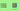# JS Challenge 2: Word ScramblesIn this article we will solve together the Scrambles challenge from CodeWars, you can find it at this link. the difficulty of this challenge is medium.

Complete the function scramble(str1, str2) that returns true if a portion of str1 characters can be rearranged to match str2, otherwise returns false.

Notes:

Only lower case letters will be used (a-z). No punctuation or digits will be included. Performance needs to be considered Input strings s1 and s2 are null-terminated.

The first example we see is this one:

``scramble('rkqodlw', 'world') ==> True``

## First Solution

My Approach for this challenge will be to iterate over the second string and create a map of how many occurrences of a character appear in it.

Let's start by doing this:

``````const count = {};

str2.split('').forEach((c) => {
count[c] = count[c] ? count[c]+=1 : 1;
})``````

I've instantiated an empty object and then I looped over the `str2` to populate it, using the letters as the keys and increasing a count to know how many times each letter appears.

We need to do this because if we didn't keep track of the count, we could end up with errors where `str1` contains all the letters from `str2` but only once, meaning it does not fulfill the requirement of being able to be re-arranged into `str2`.

Be careful, we cannot call `.forEach` on a `string`, that's why we need to first convert it into an array using `.split('')`.

Now, taking the first example and running the code against it we will get something like this:

``````{
w: 1,
o: 1,
r: 1,
l: 1,
d: 1
}``````

What we have to do now is to iterate over the first string and for each character of it, check if it appears in this Object we created. If it does, we decrease the count by 1 every time we find it.

``````str1.split('').forEach((c) => {
!!count[c] && count[c]--
});``````

Here we are doing the same as before, transforming the `string` into an `Array` and iterating over it. At each iteration, we check if the `count` has a truthy value and in that case, we reduce it by 1. We need to check first, because the second string may contain different letters altogether so it could try accessing the `Object` with properties that don't exist on it.

Once we have done this we need to check if every property of the `count` `Object` is now at 0.

``return Object.keys(count).every((key) => count[key] === 0);``

If you don't know how to use `.every` you can read more about in my article about find and replace in Array.

Putting everything together, it will look like this:

``````function scramble(str1, str2) {

const count = {};

str2.split('').forEach((c) => {
count[c] = count[c] ? count[c]+=1 : 1;
})

str1.split('').forEach((c) => {
count[c] && count[c]--;
});

return Object.keys(count).every((key) => count[key] === 0);
}``````
JS Challenge 3: Remove Zeroes

## Second Solution

Let's try now with a different solution and instead of creating a count map of the letters from `str2` let's do it with `str1`.

``````const count = {};

str1.split('').forEach((c) => {
count[c] = count[c] ? count[c]+=1 : 1;
})``````

This is the same code as before, I just replaced `str2` with `str1`.

Now, instead of mapping `str1`, reducing the count of each letter from `str2` and then checking the Object if all keys are now of value 0, we can do it a bit differently.

We can loop over `str2` and for each letter, we try to reduce the value in our `count` Object. If the action succeeds for all letters in `str2` it means that `str1` can be rearranged to form `str2`.

Let's see it in action:

``````return str2.split('').every((c) => {
return count[c]--
});``````

What this code is doing is iterating over each letter of `str2`, reducing the count each time.

We don't care if the count reached 0 or not in this case because `str1` may be much much longer than `str2`.

What we are checking here is that `return count[c]--` will not return `false` either by not finding the corresponding match or by going to a negative value which would mean that `str2` contains more occurrences of that letter than `str1`.

The complete solutions looks like this:

``````function scramble(str1, str2) {

const count = {};

str1.split('').forEach((c) => {
count[c] = count[c] ? count[c]+=1 : 1;
})

return str2.split('').every((c) => {
return count[c]--
});

}``````

There are many other ways of solving this problem, let me know yours in the comment.

If you liked this type of content, please let me know in the comments and I'll create more of these.